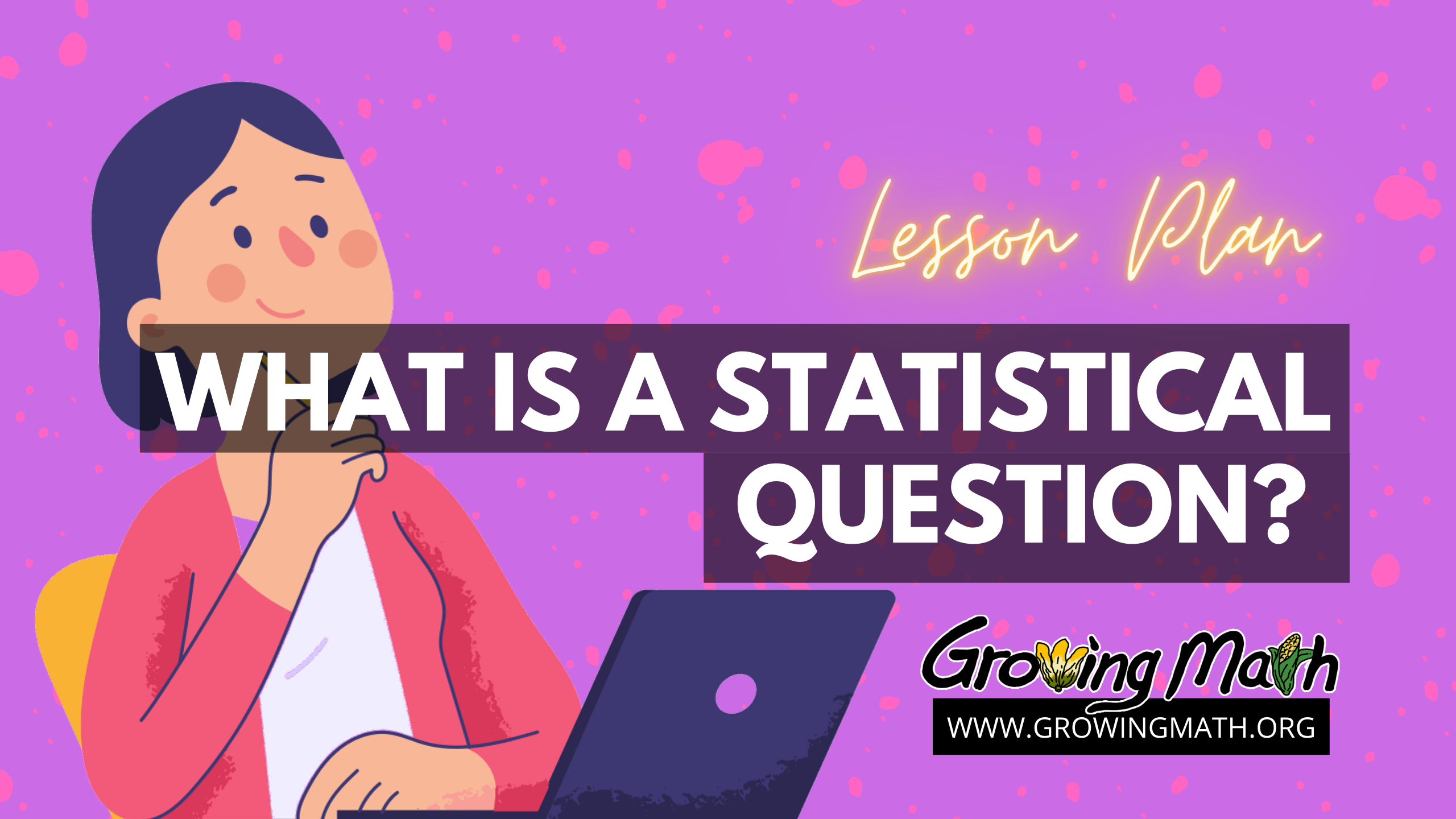# What is a statistical question?

## 📖 Standard

CCSS.MATH.CONTENT.6.SP.A.1 Recognize a statistical question as one that anticipates
variability in the data related to the question and accounts

## 📲 Technology Required

Computer with projector, for students learning in class. For students learning at home, materials can be accessed on any device with a browser and application to read PDF files or can be printed out and sent home with students.

## ⏰ Time Required

2 hours (We recommend doing this over two class periods)

## 📃 Summary

Teachers begin the lesson with a Google slides presentation explaining the requirements for a statistical question. Students complete an assignment identifying whether or not a question qualifies as a statistical question. After class discussion, students complete a second assignment using a small data set shown on a map. In Part 3, students write and answer their own statistical questions using a data set provided, giving an explanation for their answers. Optionally, students can complete a more challenging assignment drawing conclusions from a graph and/or play a game and identify statistical questions.

## 📚 Lesson

Before you begin, you should have printed out or added to your Google classroom and shared with students the STUDENT handout from the U.S. Census Bureau, “What is a statistical question?” The student version is 11 pages. If you are short on printer paper, you can skip printing page 1. Also, pages 7 and 8 are an optional activity.

You should also print or download the TEACHER version of the Census Bureau handout, “What is a statistical question?” which includes answers to questions in the first two assignments and explanations why each answer was or was not a statistical question.

### Introduce students to definition of a statistical question

Begin with the Google Slides presentation, “What is a statistical question?” which breaks down the two components of a statistical question – it must be answered by data and the data must vary.

### Students complete assignment on identifying a statistical question

Have students complete Part 1 of the handout “What is a statistical question?” After all students have answered the questions in Part 1, discuss their answers in small groups or as a class.

### Students complete Part 2, assignment on identifying a statistical question using real data

Have students complete Part 2 of the handout “What is a statistical question?” After all students have answered the questions in Part 1, discuss their answers in small groups or as a class.

Then, continue with the Google slides presentation and have students complete Part 2 of the student handout from the U.S. Census Bureau (linked above). Have students discuss their answers with one another.

Either correct the answers as a class or collect these to correct yourself. Remember, the teacher handout, linked above, has the correct answers.

We recommend you end the first day’s lesson here and begin the next lesson after students have had the assignments from Part 1 and Part 2 corrected.

### Students complete part 3, creating their own statistical questions from data.

Students complete Part 3 of the handout on “What is a statistical question?”

Discuss students’ answers in class. Provide feedback on whether a question really is a statistical question and whether students’ answers to their questions are correct. Allow students time to explain their conclusions.

### Optional: Students Complete Part 4

First, use the Google slides presentation, starting on Slide 24, to explain how to read an area chart.

Next, have students complete Part 4 from the student handout, “Drawing conclusions from a graph.”

### Optional: Play Empiric Empire

After students have completed Parts 1 to 3 of the student handout (and, optionally, Part 4), have them play the game Empiric Empire. As an additional optional assignment, ask students to identify statistical questions asked and answered during the game.

If students do not have phones but have Chromebooks, they can play Disaster Deduction Detectives instead – available June, 2022.

## Assessment

For assignments in Parts 1 and 2 the teacher version of the handout has correct answers and explanations. For assignments in Parts 3 and 4 of the student handout, examples of correct responses are given but these will vary as students provide their own statistical questions.

For the Empiric Empire game, the teacher reports show student responses to questions. It should be noted that this game does begin with fractions and decimals, which are a prerequisite to statistics.